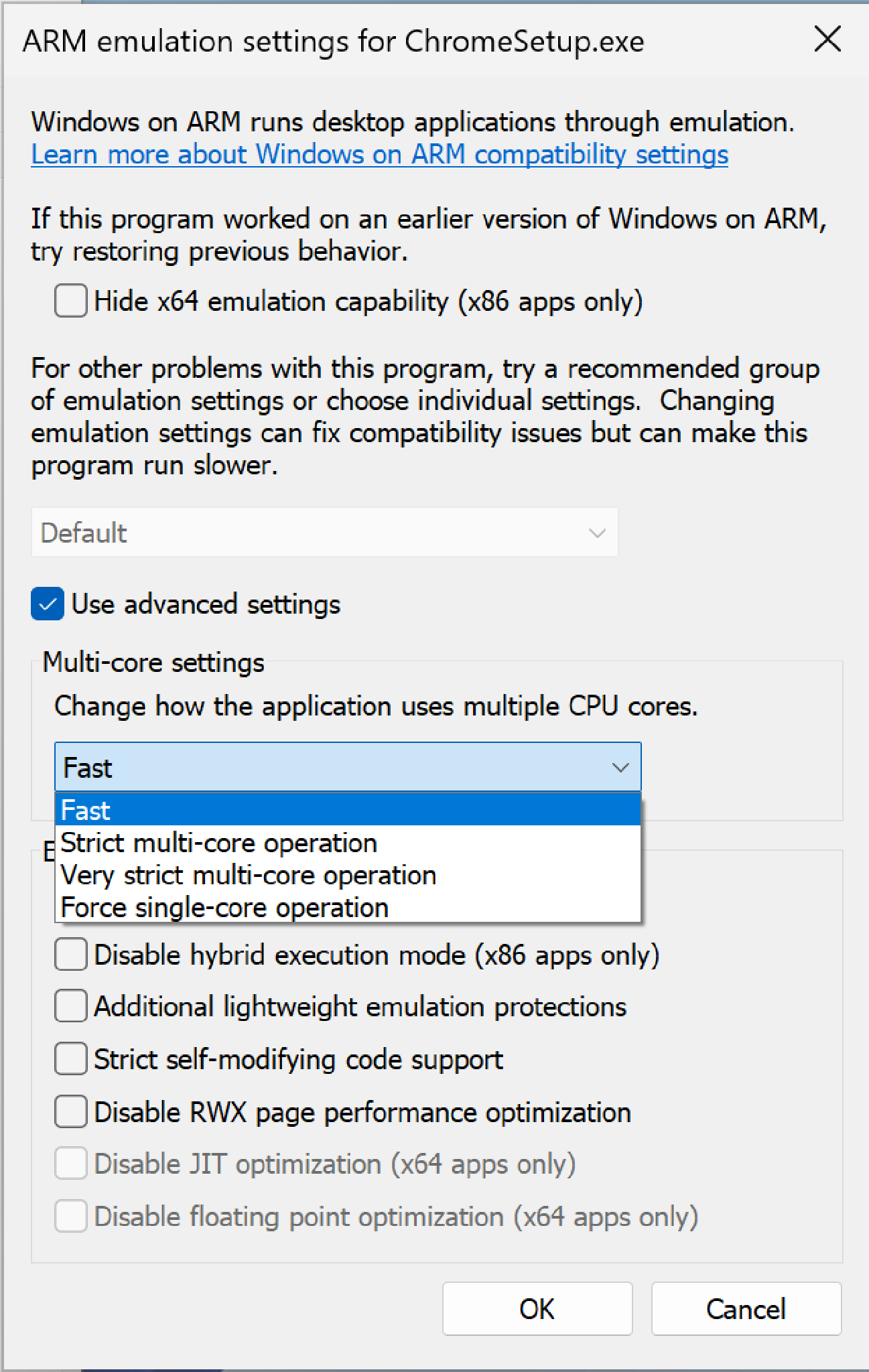# 聊聊内存序

## 概念

### Cache Coherence

Cache Coherence需要做的就是让Cache变为透明，将写操作能够eventually传播到其他处理器（也包括一个处理器核中从L1 D-Cache传播到L1 I-Cache）。

## 内存序

int x = 0;
int y = 0;

void t1() {
y = 1;
int res = x;
printf("x=%d\n",res);
}

void t2() {
x = 1;
int res = y;
printf("y=%d\n",res);
}

### Sequential Consistency

我们可以分为以下几种情况：

• If L(a) <p L(b) ⇒ L(a) <m L(b) / Load to Load /
• If L(a) <p S(b) ⇒ L(a) <m S(b) / Load to Store /
• If S(a) <p L(b) ⇒ S(a) <m L(b) / Store to Load /
• If S(a) <p S(b) ⇒ S(a) <m S(b) / Store to Store /

• L(a) = Value of MAX <m {S(a) | S(a) <m L(a)}

其中MAX <m定义为内存序中的最新。

int sum = 0;
void t1() {
sum += 1
}

void t2() {
sum += 2;
}

• x=x+1
• Store(sum,x)

aarch64基本指令集提供LDXR/SDXR等类似lr/sc的指令，自ARMv8.1起提供了LDADD等类似lock add的指令。

### Total Store Order

#### TSO定义

我们可以分为以下几种情况：

• If L(a) <p L(b) ⇒ L(a) <m L(b) / Load to Load /
• If L(a) <p S(b) ⇒ L(a) <m S(b) / Load to Store /
• If S(a) <p L(b) ⇒ S(a) <m L(b) / Store to Load /
• If S(a) <p S(b) ⇒ S(a) <m S(b) / Store to Store /

• L(a) = Value of MAX <m {S(a) | S(a) <m L(a) or S(a) <p L(a)}

int x = 0;
int y = 0;

void t1() {
y = 1;
int res = x;
printf("x=%d\n",res);
}

void t2() {
x = 1;
int res = y;
printf("y=%d\n",res);
}

#### 同步互斥怎么办？

1. RMW指令保证了访问锁本身的原子性

我们假设一个互斥锁用1表示被占用的状态，0表示自由状态，那么使用任意swap用途的RMW指令/RMW指令序列将该锁的值写为1，并判断返回值是否为0。毕竟RMW指令已经保证了整个过程只有一个Writer。

2. Peterson’s Algorithm

不了解该算法的读者可以先查阅Wikipedia

### RMC

RMC=>Relaxed Memory Consistency。

#### SC for DRF

Sequential Consistency for Data-Race-Free Programs是本书的一个很重要的概念。我们前文已经介绍了硬件提供的各式各样的内存屏障指令以及原子操作指令。在这种情况下，程序员只需要通过这些指令实现正确的同步，那么程序的执行是与SC内存序无异的。

## Causality and Write Atomicty

Causality：If I see it and tell you about it, then you will see it too.

Write Atomicity: a core’s store is logically seen by all other cores at once.

### Causality

int x = 0;
int y = 0;
void t1() {
x = 1;
}
void t2() {
y = 2;
}
void t3() {
print(x);
fence();
print(y);
}
void t4() {
print(y);
fence();
print(x);
}

## 二进制翻译

### 在RMC上运行使用TSO编写的程序

• QEMU-TCG：插入大量Barrier，性能不好但是正确性得到保证。
• Apple Rosetta 2：Apple M1硬件支持TSO模式，并可通过CSR配置，对于运行x86软件的核心将TSO开启，这样翻译时可省略不必要的Memory Barrier。这是非常高效的软硬件协同二进制翻译
• XTAJIT(Windows on ARM)：默认不插Barrier，用户如果遇到兼容性问题时提供多种选项（例如退化到单核运行或是不同等级的插入Barrier的方式运行）## One Response

1.Xavier Hsinyuan说道：